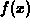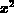Next: Three dimensional plotting Up: Getting Started With Previous: Defining Compound Functions

## Plotting Functions.

The command in Maple is plot. The syntax is

```  > plot(f(x),x=a..b,y=c..d);
```

whereis a function or an expression. You can leave out the specification of the range, but the domain must be specified. To plot several functions at a time, the syntax is

```  > plot({f(x),g(x),h(x),...},x=a..b,y=c..d);
```

Where f, g, and h are functions and ``...'' means you can plot more than three functions at a time. You can also plot expressions you have defined, or simply use expressions as arguments to the plot command. For example, you could plot the expression p defined earlier and the expressionon the same plot with the command

```  > plot(p,x^2,x=1..5);
```

You can also plot parametric curves of the formwith the Maple plot command. The example given below plots the unit circle.

```  > plot([cos(t),sin(t),t=-Pi..Pi]);
```

William W. Farr
Mon Aug 28 09:31:56 EDT 1995